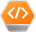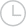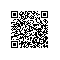# 优化WHERE子句-问答-阿里云开发者社区-阿里云

## 优化WHERE子句剑曼红尘2020-03-31 11:26:53177取消 提交回答

• 剑曼红尘2020-03-31 11:28:54

解题方法

对于此类考题，先说明如何定位低效SQL语句，然后根据SQL语句可能低效的原因做排查，先从索引着手，如果索引没有问题，考虑以上几个方面，数据访问的问题，长难查询句的问题还是一些特定类型优化的问题，逐一回答。

SQL语句优化的一些方法？

1.对查询进行优化，应尽量避免全表扫描，首先应考虑在 where 及 order by 涉及的列上建立索引。 2.应尽量避免在 where 子句中对字段进行 null 值判断，否则将导致引擎放弃使用索引而进行全表扫描，如：\

``````select id from t where num is null
-- 可以在num上设置默认值0，确保表中num列没有null值，然后这样查询：
select id from t where num=

``````

3.应尽量避免在 where 子句中使用!=或<>操作符，否则引擎将放弃使用索引而进行全表扫描。 4.应尽量避免在 where 子句中使用or 来连接条件，否则将导致引擎放弃使用索引而进行全表扫描，如：

``````select id from t where num=10 or num=20
-- 可以这样查询：
select id from t where num=10 union all select id from t where num=20
``````

5.in 和 not in 也要慎用，否则会导致全表扫描，如：

``````select id from t where num in(1,2,3)
-- 对于连续的数值，能用 between 就不要用 in 了：
select id from t where num between 1 and 3
``````

6.下面的查询也将导致全表扫描：select id from t where name like ‘%李%’若要提高效率，可以考虑全文检索。 7.如果在 where 子句中使用参数，也会导致全表扫描。因为SQL只有在运行时才会解析局部变量，但优化程序不能将访问计划的选择推迟到运行时；它必须在编译时进行选择。然 而，如果在编译时建立访问计划，变量的值还是未知的，因而无法作为索引选择的输入项。如下面语句将进行全表扫描：

``````select id from t where num=@num
-- 可以改为强制查询使用索引：
select id from t with(index(索引名)) where num=@num
``````

8.应尽量避免在 where 子句中对字段进行表达式操作，这将导致引擎放弃使用索引而进行全表扫描。如：

``````select id from t where num/2=100
-- 应改为:
select id from t where num=100*2
``````

9.应尽量避免在where子句中对字段进行函数操作，这将导致引擎放弃使用索引而进行全表扫描。如：

``````select id from t where substring(name,1,3)=’abc’
-- name以abc开头的id应改为:
select id from t where name like ‘abc%’
``````

10.不要在 where 子句中的“=”左边进行函数、算术运算或其他表达式运算，否则系统将可能无法正确使用索引。

0 0使用钉钉扫一扫加入圈子
+ 订阅